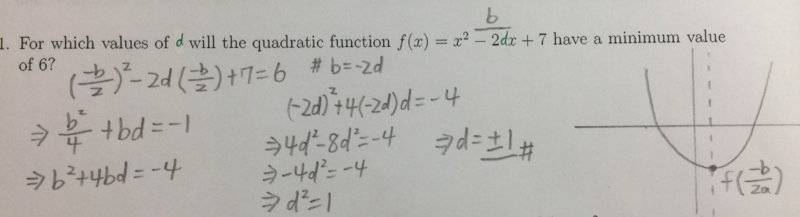# Quadratic function solution method optimizing

## Homework Statement

For which values of b will the quadratic function ƒ(x) = x2-2bx+7 have a minimum value of 6?

## Homework Equations

y = ax2+bx+c
y = a(x-h)2+k

b(first one) = -2ah(second one)
c(first one) = ah2+k(second one)

## The Attempt at a Solution

Well, I actually already have the answer to the question: b = ±1, but I believe there must be a better method to this.

My poor method goes like this:

Since the parabola has a "minimum", it must open upwards,with a vertex's y position as 6, which makes the function I am dealing with looks like these two forms:
• y = x2-2bx+7
• y = (x-h)2+6
which the second one can be turned into y = x2-2hx+h2+6
∴ h2+6 = 7(c)
∴h = ±1

then because -2b = -2h,and h = ±1, b = ±1.

Is there a simpler way of doing this?

Last edited:

verty
Homework Helper
The minimum or maximum value of a parabola is ##f({-b \over 2a})## where ##f(x) = ax^2 + bx + c##.

•scottdave and YoungPhysicist
The minimum or maximum value of a parabola is ##f({-b \over 2a})## where ##f(x) = ax^2 + bx + c##.
Thanks!

scottdave
Homework Helper
Gold Member
With a minimum value of 6, this parabola doesn't have real roots, but if we took a shifted parabola down by 6, the vertex would just touch the x axis. Thia happens when the discriminant of the quadratic formula is zero. If you subtract 6 then solve for the discriminant equal zero.

•YoungPhysicist
With a minimum value of 6, this parabola doesn't have real roots, but if we took a shifted parabola down by 6, the vertex would just touch the x axis. Thia happens when the discriminant of the quadratic formula is zero. If you subtract 6 then solve for the discriminant equal zero.
Thanks,too. Yet another great method.Ray Vickson
Homework Helper
Dearly Missed

## Homework Statement

For which values of b will the quadratic function ƒ(x) = x2-2bx+7 have a minimum value of 6?

## Homework Equations

y = ax2+bx+c
y = a(x-h)2+k

b(first one) = -2ah(second one)
c(first one) = ah2+k(second one)

## The Attempt at a Solution

Well, I actually already have the answer to the question: b = ±1, but I believe there must be a better method to this.

My poor method goes like this:

Since the parabola has a "minimum", it must open upwards,with a vertex's y position as 6, which makes the function I am dealing with looks like these two forms:
• y = x2-2bx+7
• y = (x-h)2+6
which the second one can be turned into y = x2-2hx+h2+6
∴ h2+6 = 7(c)
∴h = ±1

then because -2b = -2h,and h = ±1, b = ±1.

Is there a simpler way of doing this?

•